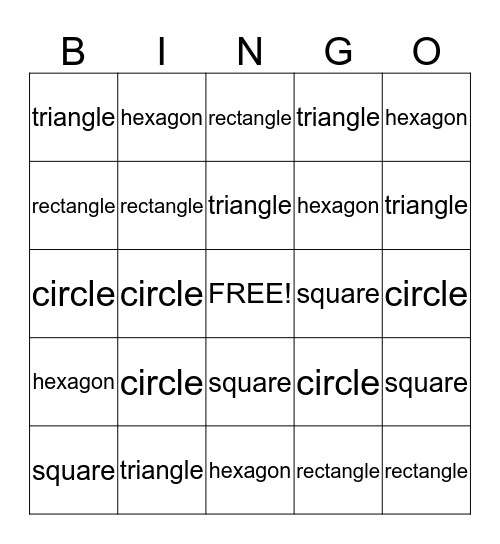# ShapesThis bingo card has a free space and 24 words: square, circle, triangle, rectangle, hexagon, triangle, hexagon, rectangle, square, circle, circle, rectangle, circle, square, rectangle, triangle, hexagon, triangle, triangle, hexagon, circle, square, hexagon and rectangle.

⚠ This card has duplicate items: square (4), circle (5), triangle (5), rectangle (5), hexagon (5)

## Play Online